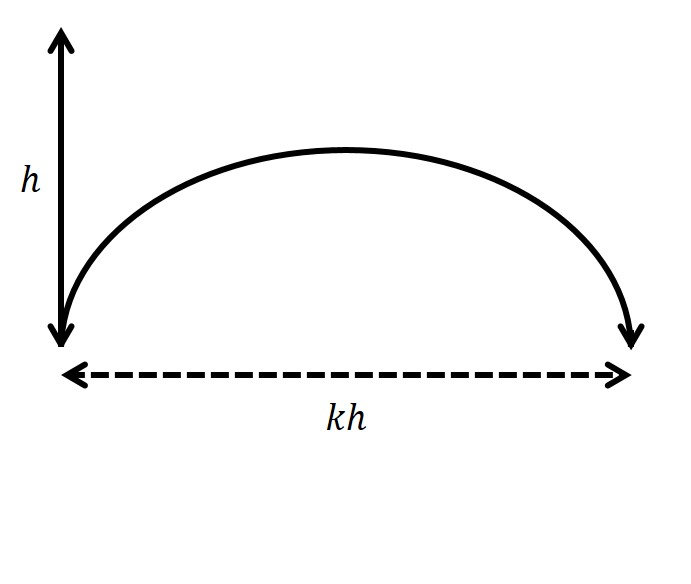# Projectile Puzzle

The maximum horizontal distance you can throw an object before it hits the ground is given by $kh$, where $k$ is a constant and $h$ is the maximum height the object reaches if thrown directly upwards at the same initial speed.What is the value of $k$?

Details and Assumptions

• There is no air resistance.

• The object is thrown from ground level in both cases.

×